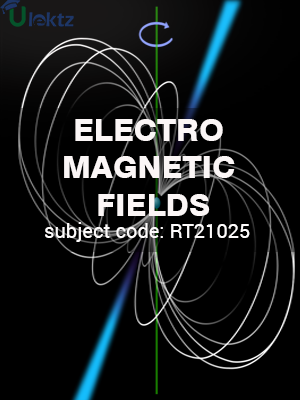uLektz apps

•My WalletMy Order
•My Profile
•My Connections
•My Books
•My Videos
•My Tests
•My Calender
•My Messages
•My Shopping Cart
•My Orders
•Account Settings
•Help

uLektz apps

Book DetailsElectromagnectic Fields

Course Code:RT21025

Author:uLektz

University:

Regulation:2013

Categories:Electrical & Electronics

Format :ePUB3 (DRM Protected)

Type :eBook

FREE

Description :Electromagnectic Fields of RT21025 covers the latest syllabus prescribed by JNTU Kakinada for regulation 2013. Author: uLektz, Published by uLektz Learning Solutions Private Limited.

Note : No printed book. Only ebook. Access eBook using uLektz apps for Android, iOS and Windows Desktop PC.

Topics
Unit – I Electrostatics

1.1 Electrostatic Fields – Coulomb’s Law

1.2 Electric Field Intensity (EFI) – EFI due to a line and a surface charge – Work done in moving a point charge in an electrostatic field

1.3 Electric Potential – Properties of potential function – Potential gradient

1.4 Guass’s law –– Maxwell’s first law, div ( D )=ρv Laplace’s and Poison’s equations and Solution of Laplace’s equation in one variable

Unit – II Conductors – Dielectrics and Capacitance

2.1 Electric dipole – Dipole moment – potential and EFI due to an electric dipole

2.2 Torque on an Electric dipole in an electric field – Behaviour of conductors in an electric field – Conductors and Insulators Polarization

2.3 Boundary conditions between conduction to Dielectric and dielectric to dielectrics capacitance – capacitance of parallel plates, spherical and coaxial cables with composite dielectrics

2.4 Energy stored and energy density in a static electric field – Current density – conduction and Convection current densities

2.5 Ohm’s law in point form – Equation of continuity

Unit – III Magneto statics and Ampere’s Law

3.1 Static magnetic fields – Biot-Savart’s law – Oesterd’s experiment

3.2 Magnetic field intensity (MFI) – MFI due to a straight current carrying filament – MFI due to circular, square and solenoid current – Carrying wire

3.3 Relation between magnetic flux, magnetic flux density and MFI – Maxwell’s second Equation, div(B)=0

3.4 Ampere’s circuital law and its applications viz. MFI due to an infinite sheet of current and a long filament carrying conductor – Point form of Ampere’s circuital law

3.5 Field due to a circular loop, rectangular and square loops, Maxwell’s third equation, Curl (H)=J.

Unit – IV Force in Magnetic fields

4.1 Magnetic force - Moving charges in a Magnetic field – Lorentz force equation

4.2 Force on a straight and a long current carrying conductor in a magnetic field – Force between two straight long and parallel current carrying conductors

4.3 Magnetic dipole and dipole moment – a differential current loop as a magnetic dipole

4.4 Torque on a current loop placed in a magnetic field

Unit – V Self and Mutual inductance

5.1 Self and Mutual inductance

5.2 Determination of self-inductance of a solenoid and toroid and mutual inductance between a straight long wire and a square loop wire in the same plane

5.3 Energy stored and density in a magnetic field.

Unit – VI Time Varying Fields

6.1 Time varying fields – Faraday’s laws of electromagnetic induction – Its integral and point forms

6.2 Maxwell’s fourth equation, Curl (E)=-∂B/∂t – Statically and Dynamically induced EMFs – Simple problems -Modification of Maxwell’s equations for time varying fields – Displacement current

6.3 Poynting Theorem and Poynting vector

Related Books